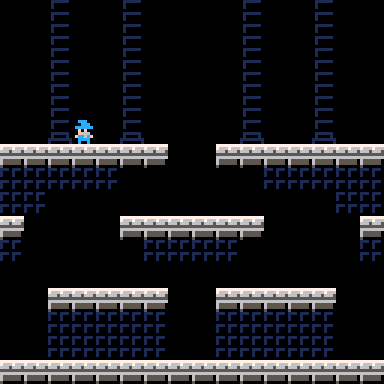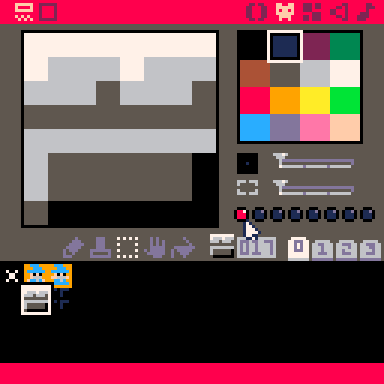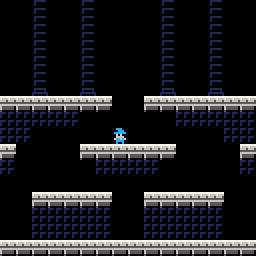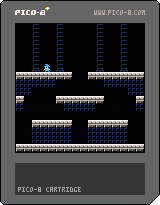# Simple Platforming

One of the surprising things about building Barrier Blast for GMTK Jam was how little time I ended up spending on the basic platformer movement. In the past I've struggled with the code for platformers. I've found it very difficult to handle collision correctly and get the physics feeling correct.

I've pulled out the code into a commented demo cart. Code on this page is free to use in your own projects.``````function _init()
-- Player X and Y position on screen.
plx = 24
ply = 40
-- Player "facing". +1 if the player is facing right, -1 if the player
-- is facing left.
plf=1
-- Boolean indicating whether the player is touching the ground. This is
-- used to make sure the player can only jump if they're standing on the
-- ground. If you don't have that check then the player can do infinite
-- jumps in midair.
plgnd=true
-- Player's y velocity. For this demo we don't use any acceleration in
-- the x direction, so we don't need to store x velocity. But we do have
-- gravity accelerating the player downward, so we need y velocity.
plvy=0
-- Boolean indicating whether the player is walking. This will be used
-- by the drawing code to decide whether it should do the walk animation.
plw=false

-- Global frame counter. This is a variable I use a lot for effects and
-- animation. In this demo it's used for the player's walk animation.
f=0
end

function _update()
-- Global frame counter gets updated each frame. By doing a modulo on this
-- frame counter it's easy to make events happen every `n` frames.
f+=1

-- Here we save the x position before we do any movement. The way we will
-- handle collisions is to try and move, check collision, and then move
-- back if we're colliding with something.
local oldx = plx

-- `dx` represents the change in the x position this frame. It's basically
-- the speed. Depending on whether the player is pressing left or right,
-- we'll add or subtract this number from the x position.
local dx=1.5

-- If the player is holding left, subtract the speed from the x position.
-- `plf` is updated to -1, which means the player is facing left. The draw
-- code will read `plf` to decide whether to flip the sprite or not.
if btn(0) then
plx-=dx
plf=-1
end

-- If the player is holding right, add the speed to the x position, and
-- update `plf` to say the player is facing right.
if btn(1) then
plx+=dx
plf=1
end

-- Note that if neither left or right is held, then we never update `plx`,
-- so the player does not move left or right on screen. We also don't
-- change `plf`, so the player will keep facing the same direction they
-- were facing in the last frame.

-- We've moved the player, now we check for collision. `sprcoll` is
-- "sprite collision". The definition is below, and we'll see that it
-- checks to see if the player is colliding with any of the walls or
-- floors drawn in the map.
if sprcoll(plx,ply) then
plx=oldx
end

-- `plw` is true if the player is walking, and false if they're not.
-- If the player is walking, the player's new x position will be
-- different from the position last frame.
plw=plx~=oldx

-- Jumping! We use `btnp` to see if the player has pressed jump _this
-- frame_. We also check `plgnd` to see if the player is touching the
-- ground.
if btnp(4) and plgnd then
-- To make the player jump, set the velocity to a negative value. No
-- acceleration.
plvy = -7
-- The player will be jumping a lot. So we add a small random chance
-- to play a different sound effect.
if rnd()<0.1 then
sfx(2)
else
sfx(1)
end
else
-- If the player isn't jumping, then we apply gravity as normal.
-- We increase the player's velocity by 1 per frame, and cap it
-- at a maximum fall speed of 4.
plvy += 1
if plvy > 4 then
plvy = 4
end
end

-- Now we will process the y movement. We are going to move 1 pixel at a
-- time, checking for collisions at each step.
-- We'll start by setting plgnd to false. If we finish moving and never
-- touch the ground then this will stay false. If we do hit the ground
-- this will be set to true and the player will be able to jump again
-- next frame.
plgnd=false
-- `mv` is the number of pixels we have to move this frame.
local mv=plvy
while abs(mv)>=1 do
-- This is the same as what we did for x movement. We'll save the
-- old position so we can back up if we hit something.
local oldy=ply
-- `sgn` gets the sign of the variable. So these lines will move the
-- player by one pixel in the correct direction, and move `mv` towards
-- zero.
ply += sgn(mv)
mv -= sgn(mv)
-- The collision check, same as for x.
if sprcoll(plx,ply) then
-- If we collided _and_ we are moving downwards, the player is now
-- standing on the ground.
if plvy>0 then
plgnd=true
end
-- We collided so we move back to oldy, and cancel out any speed.
-- We also stop the loop here because movement is done.
ply=oldy
plvy=0
break
end
end

-- Screen wraparound. We just move the player to the other side if they go
-- off either end of the screen.
if plx>128 then
plx=-8
end
if plx<-8 then
plx=128
end
if ply<-8 then
ply=128
end
if ply>128 then
ply=-8
end
end

-- So that's movement, but collision detection was hidden in the `sprcoll`
-- function. `sprcoll` is still pretty simple. It checks the 4 corners of the
-- sprite at (x,y) to see if they are blocked by tiles on the map. We assume
-- the sprite is 8 by 8. I also fudged the numbers in the x direction a little
-- bit to make the collision rectangle a little narrower to match up with the
-- player's sprite. So if you use this you might need to change up the points
-- here to fit your sprite.
function sprcoll(x,y)
return solid(x+1,y)
or solid(x+6,y)
or solid(x+1,y+7)
or solid(x+6,y+7)
end

-- And here is how we check if a pixel is solid. `i` and `j` represent the
-- coordinates of this pixel on the map. We get this by dividing by 8, and then
-- we use a modulo to wrap the coordinates around. If the player is close to
-- one edge of the screen, then one of the points we check for collision may
-- be over the edge of the map. If the player is off the left side of the
-- screen, then the check might use a negative number. Fortunately the way
-- PICO-8 handles modulo for negative numbers works out in our favor.
function solid(x,y)
local i=(x/8)%16
local j=(y/8)%16
-- First we get the sprite at that map location, and then we check
-- one of the `flags` on that sprite. Flags are super handy for when
-- you have a bunch of different map tiles that need to share some
-- property, like being solid.
--
-- To set a flag, find the sprite for your tile in the sprite editor, and
-- select one of the small circles below the color palette. The circle
-- will light up when the flag is set to 1. The flags are numbered starting
-- with 0 on the left.
local s=mget(i,j)
return fget(s,0)
end

function _draw()
cls()

-- First we draw the room from the map.
map(0,0,0,0,16,16)

-- Our sprite has black pixels, so I used orange for transparency. These
-- two calls turn off transparency for black and turn it on for orange.
palt(0,false)
palt(9,true)

-- `s` is the sprite we'll draw, and `y` is the position we'll draw the
-- player at. Both of these will be affected by the animation.
local s=1
local y=ply

-- If the player is not on the ground, we'll always draw the "jumping"
-- version of the sprite.
if not plgnd then
s=2
-- If the player is walking we figure out which frame of the walk cycle to
-- draw.
elseif plw then
-- A modulo operation on the global frame counter is a quick way to do
-- the timing for the animation. Every 6 frames we switch the sprite.
if f%12>6 then
-- We switch to the "jumping" sprite and move the sprite up a pixel
-- to make it look like the wizard is hopping along.
y-=1
s=2
end
end
-- Draw the sprite, using the results from the animation logic.
-- We also check plf to see if the sprite needs to be flipped.
spr(s,plx,y,1,1,plf<0)

-- Reset the palette, so that the transparency changes we made don't affect
-- future draw calls.
pal()
end
``````